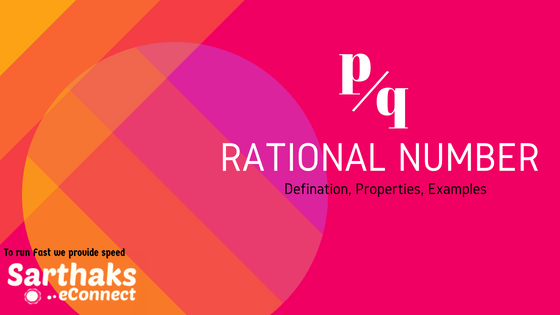We have learnt counting numbers (1,2,3,4….). Then we got to know about natural numbers which are the same as counting numbers. Than whole numbers which are the natural number along with zero (0,1,2,3,4…). When our need increased we learn integers (….-3,-2,-1,0, 1, 2, 3, ….). For representing directional distance as +5km i.e 5km forward and -5km i.e. 5km backwards. There are much more applications of integers as in profit loss. Then we knew about fractions which is $$\frac{numerator}{denominator}$$ . Thus we needed Rational number.

# Rational Number:

Again we needed a term which can be used in various circumstances as in different form of quantities. As when we have to represent a distance of 500m we write it in the form of $$\frac{1}{2}$$ km. Also you can’t wite 500m as only $$\frac{1}{2}$$ as fraction but you have to write with denomination i.e $$\frac{1}{2}$$ km.

# Definition:

The word ‘rational’ arises from the term ‘ratio’. You know that a ratio like 1:2 can also be written as $$\frac{1}{2}$$. Here, 1 and 2 are natural numbers.

A number which can be represented in the form of $$\frac{p}{q}$$ where p and q are integers and q≠0.

Example: In $$\frac{1}{2}$$, p = 1, q = 2 ≠ 0, thus, $$\frac{1}{2}$$ represents a rational  no.

Rational number includes Integers and fractions.

## Equivalent Rational Number:

A rational no. can be represented with different numerators and denominators.

We can write $$\frac{1}{2}$$ = $$\frac{2}{4}$$ = $$\frac{3}{6}$$ and so on by simply multiplying or dividing numerator and denominator by the same non-zero number.

## Positive Rational Number:

When both the numerator and denominator of any rational fractional number are positive integers.

As, In $$\frac{4}{5}$$ both 4 and 5 are positive integers thus is a positive rational no.

## Negative Rational Number:

When the numerator is a negative integer, whereas the denominator is a positive integer.

As In -$$\frac{5}{7}$$ both 4 is negative integer and 5 is positive integer thus is a negative rational no.

“The number 0 is neither a positive nor a negative rational number.”

## Standard Form of Rational Number:

A rational number is said to be in the standard form if its denominator is a positive integer and the numerator and denominator have no common factor other than 1.

As, $$\frac{1}{2}$$, $$\frac{4}{5}$$, $$\frac{5}{7}$$ are in standard form.

## Properties of rational number:

• There is infinite number of rational numbers between two rational numbers.
• Two rational fractional numbers with the same denominator can be added by adding their numerators, keeping the denominator same.
• Two rational fractional numbers with different denominators are added by first taking the LCM of the two denominators and then converting both the rational numbers to their equivalent forms having the LCM as the denominator.
• While subtracting two rational numbers, we add the additive inverse of the rational fraction to be subtracted to the other rational fraction.
• To multiply two rational numbers, we multiply their numerators and denominators separately and write the product as $$\frac{product \:of\:numerators}{product \:of \:denominators}$$.
• To divide one rational number by the other non-zero rational number, we multiply the rational number by the reciprocal of the other.

## Example:

1. Reduce $$\frac{40}{30}$$ to standard form.

Solution:
We first divide the numerator and denominator by a common divisible number

$$\frac{40}{30}$$ = $$\frac{40÷10}{30÷10}$$ = $$\frac{4}{3}$$
We divided the numerator and denominator by 10 and 10 is also the HCF of the two number.

Thus, to reduce the rational fraction to its standard form, we divide its numerator and denominator by their HCF ignoring the negative sign, if any.

1. List three rational numbers between 1 and 2.

Solution:
Let us write 1 and 2 as rational numbers with denominator 4.

1 = $$\frac{4}{4}$$ and 2 = $$\frac{8}{4}$$
The rational number between $$\frac{4}{4}$$ and $$\frac{8}{4}$$ are

$$\frac{5}{4}$$, $$\frac{6}{4}$$, $$\frac{7}{4}$$